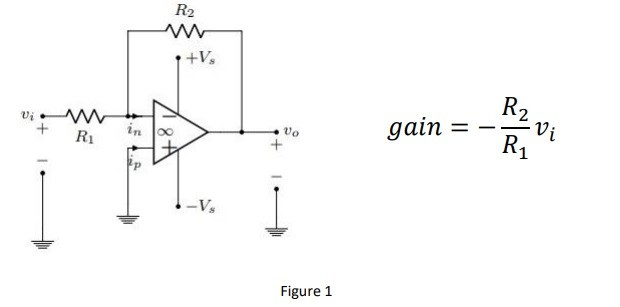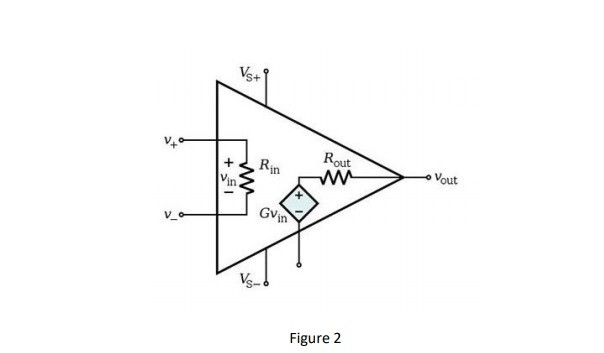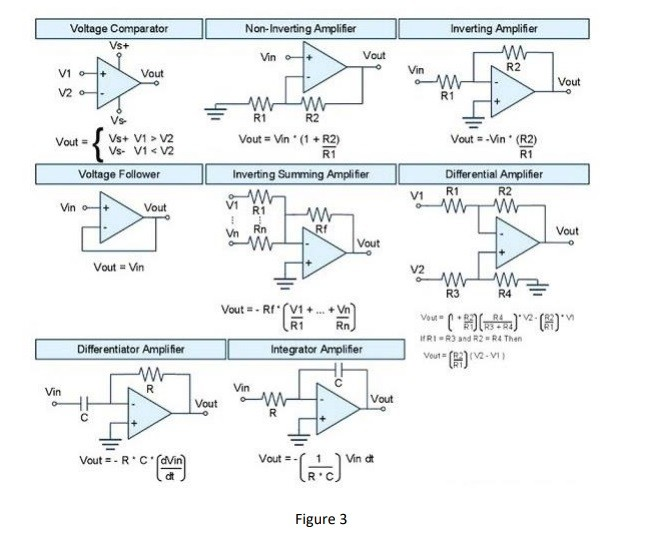YazarHakan Uzunöz
657 görüntülenme
1 dakika okuma süresi
May 13, 2019

## op-amps

What is the op-amp

Op-amp or operational amplifier is a high voltage gain electronic signal amplifier. A opamp

have 4 input and 1 output generally. The inputs are inverting input, non-inverting input and

+V source, -V source inputs. It is linear device and using filtering or perform mathematical

operations (add, subtract, integration and differentiation). Multipliers of opamps can change

ambient conditions easly so we should use feedback resistors or capacitors between input

and output. Also we can identify gain with the ratio of feedback resistors. The figure 1 shows

us the connection and formula of gain.This connection called negative feedback and we can protect our circuit from environmental

factors for example; temperature.

Figure 2 shows us the internal structure of opamp. When we idealize the opamp agree the

Rin = ∞ , A=∞ and Rout=0. Also the figure 3 is type of opamps